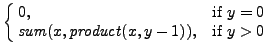Next: Example: recursive implementation of Up: Unit 11 Previous: Example: recursive implementation of

## Example: recursive implementation of the product between two integers

We exploit the following recursive definition of the product between two non-negative integers:

product(x, y) =Implementation (we assume that the method sum() is defined in the same class):

public static int product(int x, int y) {
if (y == 0)
return 0;
else
return sum(x, product(x, y-1));
}Next: Example: recursive implementation of Up: Unit 11 Previous: Example: recursive implementation of